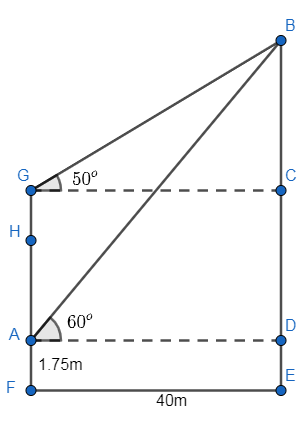QUESTION

# A 1.75 metre tall man, standing at the foot of a tower, sees the top of a hill 40 metres away at an elevation of ${{60}^{\circ}}$. Climbing to the top of the tower, he sees it at an elevation of ${{50}^{\circ}}$. Calculate the heights of the tower and the hill.

Hint: Draw the figure then use the trigonometric ratio and relate it with sides of the triangle. Then try to find the height of tower and hill using the data of the length which is given between the tower and the hill.

In the question a 1.75m tall man who is standing at the foot of the tower, sees at the top of the hill 40m away at an elevation of ${{60}^{\circ}}$. Now he climbed at the top of the tower and he saw at the top of the hill with an elevation of ${{50}^{\circ}}$.
Let us draw the figure first,We can write or represent AF as the height of man, FG as height of the tower and BE as the height of the hill, EF and AD as the distance between tower and the hill.
So, let $BE=x$. From figure we see that,
BE = BD + DE
But DE = 1.75, so substituting the corresponding values, we get
\begin{align} & x=BD+1.75 \\ & \Rightarrow BD=x-1.75........(i) \\ \end{align}
Now let us consider $\Delta BAD$, applying the trigonometric ratios, we get
$\tan \left( \angle BAD \right)=\dfrac{BD}{DA}$
Now it is given $\angle BAD={{60}^{\circ}}$ andusing values from equation (i) and figure, we get
$\tan \left( {{60}^{\circ}} \right)=\dfrac{x-1.75}{40}$
Now by using the value of $\tan \left( {{60}^{\circ}} \right)=\sqrt{3}$, we get
\begin{align} & \sqrt{3}=\dfrac{x-1.75}{40} \\ & \Rightarrow x-1.75=40\sqrt{3} \\ & \Rightarrow x=40\sqrt{3}+1.75 \\ \end{align}
Substituting the value of $\sqrt{3}$, we get
\begin{align} & \Rightarrow x=40\left( 1.732 \right)+1.75 \\ & \Rightarrow x=69.28+1.75=71.03m \\ \end{align}
So, the value of BE is 71.03m.
Now let us consider $\Delta BGC$, applying the trigonometric ratios, we get
$\tan \left( \angle BGC \right)=\dfrac{BC}{GC}$
As we know, $\angle BGC={{50}^{\circ}}$, from figure GC is the distance between the tower and the hill, so
$\tan \left( {{50}^{\circ}} \right)=\dfrac{BC}{40}$
By table of trigonometric table, we say that $\tan \left( {{50}^{\circ}} \right)=1.19$, so the above ratio becomes,
\begin{align} & 1.19=\dfrac{BC}{40} \\ & \Rightarrow BC=1.19\times 40=47.6m \\ \end{align}
From figure, we see that
$FG=BE-BC$
Substituting the corresponding values, we get
$FG=71.03-47.06=23.43m$
So, the height of the tower is 23.43m and the height of the hill is 71.03m.

Note: Students should know how to represent trigonometric ratios as the sides of a right angled triangle. They should also be careful about the calculations to avoid any errors.
Another approach is, if the value of $\tan \left( {{50}^{\circ}} \right)=1.19$ is not known, then we can apply trigonometric identities formulas, to calculate this.